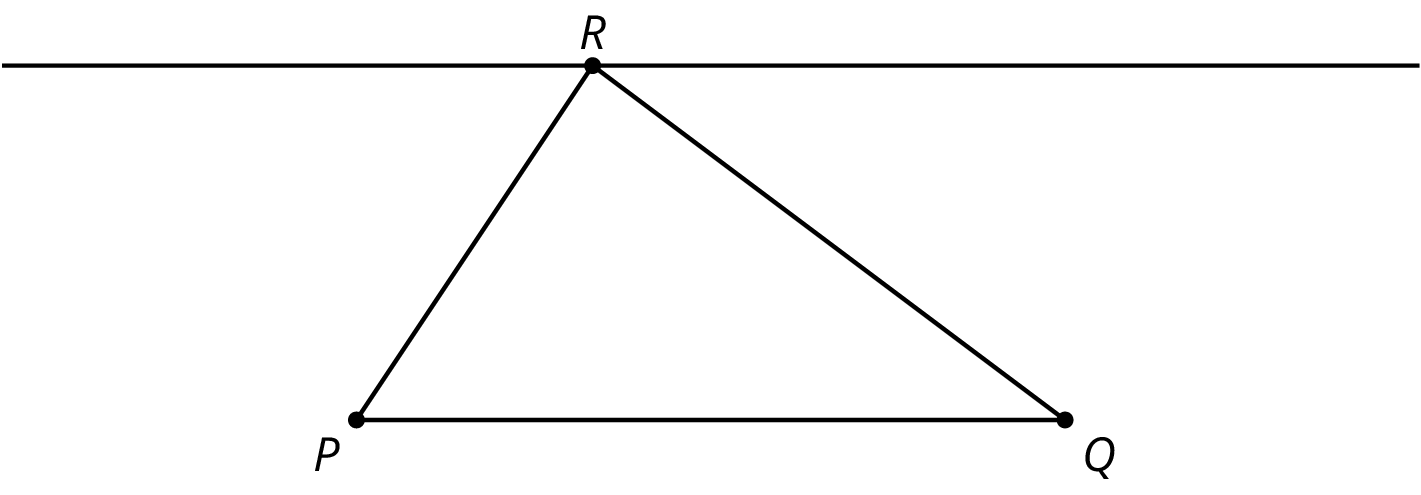Lesson plan

# Lesson 16: Parallel Lines and the Angles in a Triangle

teaches Alabama State Standards 8-25.a.
teaches Alabama State Standards 8-25.
teaches Arizona State Standards 8.G.A.5
teaches Common Core State Standards MP7 http://corestandards.org/Math/Practice/MP7
teaches Common Core State Standards 8.G.A.5 http://corestandards.org/Math/Content/8/G/A/5
teaches Georgia State Standards MGSE8.G.5.
teaches Kansas State Standards 8.G.5.
teaches New York State Standards NY-8.G.5.
teaches Ohio State Standards 8.G.5.
teaches Pennsylvania State Standards CC.2.3.8.A.2.

# Lesson 16: Parallel Lines and the Angles in a Triangle

Earlier in this unit, students learned that when a $$180^\circ$$ rotation is applied to a line $$ℓ$$, the resulting line is parallel to $$ℓ$$. Here is a picture students worked with earlier in the unit:The picture was created by applying $$180^\circ$$ rotations to $$\triangle ABC$$ with centers at the midpoints of segments $$AC$$ and $$AB$$. Notice that $$E$$$$A$$, and $$D$$ all lie on the same grid line that is parallel to line $$BC$$. In this case, we have the structure of the grid to help see why this is true. This argument exhibits one aspect of MP7, using the structure of the grid to help explain why the three angles in this triangle add to 180 degrees.In this lesson, students begin by examining the argument using grid lines described above. Then they examine a triangle off of a grid, $$PQR$$. Here an auxiliary line plays the role of the grid lines: the line parallel to line $$PQ$$  through the opposite vertex $$R$$. The three angles sharing vertex $$R$$ make a line and so they add to 180 degrees. Using what they have learned earlier in this unit (either congruent alternate interior angles for parallel lines cut by a transverse or applying rigid transformations explicitly), students argue that the sum of the angles in triangle $$PQR$$ is the same as the sum of the angles meeting at vertex $$R$$. This shows that the sum of the angles in any triangle is 180 degrees. The idea of using an auxiliary line in a construction to solve a problem is explicitly called out in MP7.

Lesson overview

• 16.1 Warm-up: True or False: Computational Relationships (5 minutes)
• 16.2 Activity: Angle Plus Two (15 minutes)
• There is a digital applet in this activity.
• 16.3 Activity: Every Triangle in the World (15 minutes)
• Includes "Are you Ready for More?" extension problem
• 16.4 Optional Activity: Four Triangles Revisited (5 minutes)
• Lesson Synthesis
• 16.5 Cool-down: Angle Sizes (5 minutes)

Learning goals:

• Create diagrams using 180-degree rotations of triangles to justify (orally and in writing) that the measure of angles in a triangle sum up to 180 degrees.
• Generalize the Triangle Sum Theorem using rigid transformations or the congruence of alternate interior angles of parallel lines cut by a transversal.

Learning goals (student facing):

• Let’s see why the angles in a triangle add to 180 degrees.

Learning targets (student facing):

• I can explain using pictures why the sum of the angles in any triangle is 180 degrees.

Required materials:

• geometry toolkits

Glossary:

• Access the complete Grade 8 glossary.

Standards

• This lesson builds on the standards: CCSS.7.NS.ACCSS.8.G.A.1.bMS.8.G.1b
• This lesson builds towards the standard: CCSS.8.G.B.6MS.8.G.6

IM 6–8 Math was originally developed by Open Up Resources and authored by Illustrative Mathematics, and is copyright 2017-2019 by Open Up Resources. It is licensed under the Creative Commons Attribution 4.0 International License (CC BY 4.0). OUR's 6–8 Math Curriculum is available at https://openupresources.org/math-curriculum/.